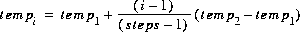[Next] [Previous] [Up] [Top]

Keywords

### REACTION_TEMPERATURE

This keyword data block is used to define temperature during reaction steps. It is necessary to enter this data block if a temperature other than the default temperature is needed for reaction calculations.

#### Example 1

```Line 0: REACTION_TEMPERATURE 1 Three explicit reaction temperatures.
Line 1:     15.0     25.0     35.0
```

#### Explanation 1

Line 0: REACTION_TEMPERATURE [number] [description]

REACTION_TEMPERATURE is the keyword for the data block.

number--positive number to designate this temperature data. Default is 1. A range of numbers may also be given in the form m-n, where m and n are positive integers, m is less than n, and the two numbers are separated by a hyphen without intervening spaces.

description--optional character field that describes the temperature data.

Line 1: list of temperatures

list of temperatures--a list of temperatures, in Celsius, that will be applied to reaction calculations. More lines may be used to supply additional temperatures. At least one reaction calculation will be performed with each listed temperature. If more reaction steps are defined in REACTION input than temperature steps in REACTION_TEMPERATURE, then the final temperature will be used for all of the additional reaction steps. If more temperature steps are defined, the final reaction step will be used for any remaining temperature steps.

#### Example 2

```Line 0: REACTION_TEMPERATURE 1 Three implicit reaction temperatures.
Line 1:      15.0     35.0 in 3 steps
```

#### Explanation 2

Line 0: REACTION_TEMPERATURE [number] [description]

Same as example 1.

Line 1: temp1, temp2, in steps

temp1--temperature of first reaction step, in Celsius.

temp2--temperature of final reaction step, in Celsius.

in steps--"in" indicates that the temperature will be calculated for each of steps number of steps. The temperature at each step, i, will be calculated by the formula. Example 2 performs exactly the same calculations as example 1. If more than steps reaction steps are defined by REACTION input, the temperature of the additional temperature steps will be temp2. If more temperature steps are defined, the final reaction step will be used for any remaining temperature steps.

#### Notes

The default temperature of a reaction step is equal to the temperature of the initial solution or the mixing-fraction-averaged temperature of a mixture. REACTION_TEMPERATURE input can be used even if there is no REACTION input. The implicit method of calculation of temperature steps is slightly different than the implicit calculation of reaction steps. If n implicit reaction steps are defined, then the reaction is added in n equal increments. If n implicit temperature steps are defined, then the temperature of the first reaction step is equal to temp1; temperatures in the remaining steps are defined by n-1 equal increments.

#### Example problems

The keyword REACTION_TEMPERATURE is used in example problem 2.

#### Related keywords

REACTION.

Example 1
Explanation 1
Example 2
Explanation 2
Notes
Example problems
Related keywords

User's Guide to PHREEQC - 07 MAY 96
[Next] [Previous] [Up] [Top]